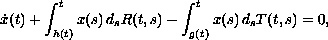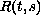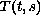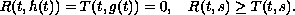Electron. J. Diff. Eqns., Vol. 2003(2003), No. 12, pp. 1-21.

### Oscillation for equations with positive and negative coefficients and with distributed delay I: General results Leonid Berezansky & Elena Braverman

Abstract:
We study a scalar delay differential equation with a bounded distributed delay,where,are nonnegative nondecreasing infor any,We establish a connection between non-oscillation of this differential equation and the corresponding differential inequalities, and between positiveness of the fundamental function and the existence of a nonnegative solution for a nonlinear integral inequality that constructed explicitly. We also present comparison theorems, and explicit non-oscillation and oscillation results.
In a separate publication (part II), we will consider applications of this theory to differential equations with several concentrated delays, integrodifferential, and mixed equations.

Submitted October 29, 2002. Published February 11, 2003.
Math Subject Classifications: 34K11, 34K15.
Key Words: Oscillation, non-oscillation, distributed delay, comparison theorems.

Show me the PDF file (240K), TEX file, and other files for this article.

A related article has been published by the same authors in this journal: Oscillation for equations with positive and negative coefficients and with distributed delay II: Applications, Vol. 2003(2003), No. 47, pp. 1-25.Leonid Berezansky Department of Mathematics Ben-Gurion University of the Negev Beer-Sheva 84105, Israel e-mail: brznsky@cs.bgu.ac.il Elena Braverman Department of Mathematics and Statistics University of Calgary 2500 University Drive N. W., Calgary, Alberta, Canada, T2N 1N4 Fax: (403)-282-5150, phone: (403)-220-3956 email: maelena@math.ucalgary.ca